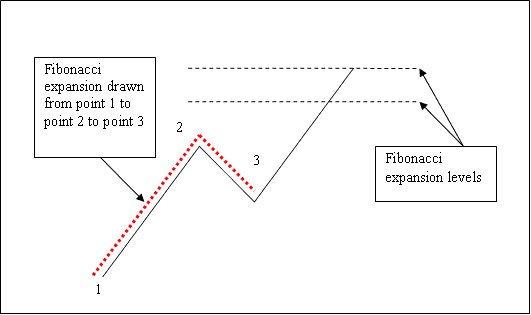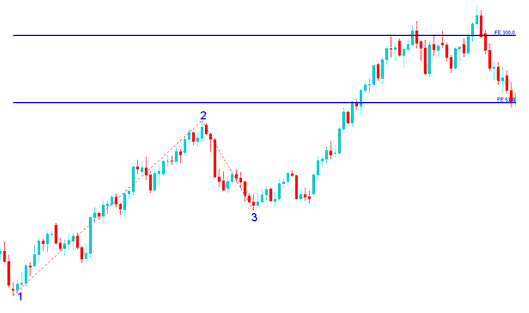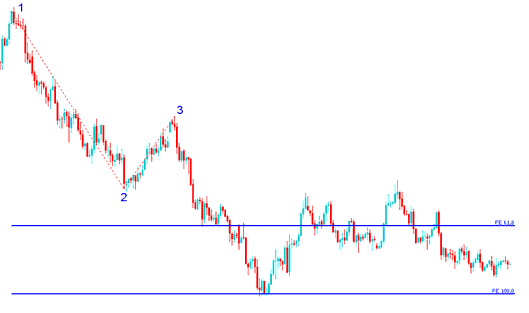# Indices Trading Fibonacci Extension Strategy using Indices Trading Fibonacci Extension Levels

Fibonacci extension levels indicator is drawn using 3 chart points.

To draw Indices Trading Fibonacci Extension levels we wait until the stock indexes price retracement is complete and the stock indexes price starts to move in the original direction of the Indices trend. Where the retracement reaches is used as chart point 3.

The Fibonacci extension example explained and illustrated below shows the 3 Chart Points where the Fibonacci extension indicator is drawn, marked as Chart point 1, 2 and 3. Chart point 1 is where the indices trend started, Chart point 2 is where the indices trend pulled back and retraced and Chart point 3 is where the stock indices retracement reached as shown on the Indices Trading Fibonacci Extension Indicator example explained and illustrated below.Please note where these Indices Trading Fibonacci Extension levels are drawn - Indices Trading Fibonacci Extension levels are drawn above the Fibonacci Indicator, these are the points where the indices trader will set the take profit orders using these Indices Trading Fibonacci Extension Levels - 61.8% and 100% Indices Trading Fibonacci Extension Levels.

## Drawing Indices Trading Fibonacci Extension Levels on an Upward Indices Trend

We use Fibonacci extensionlevels to estimate where the indices trend movement will reach. There are 2 important Fibonacci extension levels; 61.8% and 100% Indices Trading Fibonacci Extension Levels, these are used for taking profit.

On the Fibonacci extension example explained and illustrated below you can see that the Fibonacci extension indicator is drawn along the direction of the indices trend, since the indices trend is upwards - the Fibonacci extension is also drawn upwards.

These Fibonacci extension levels are displayed as horizontal lines above the Indices Trading Fibonacci Extension indicator, showing profit taking areas. In the stock indexes trading example explained and illustrated below if you had used of 100% Fibonacci extension you would have made nice profit from the trade setup.Drawing Indices Trading Fibonacci Extension Levels on an Upward Indices Trend - Indices Trading Fibonacci Extension Strategies in Indices Trading

From the above Fibonacci extension examples, the upward indices trend continued and both 61.8% and 100.0% Fibonacci extension levels were all hit after which stock indexes price retraced again after hitting the 100.0 % Indices Trading Fibonacci Extension level.

## Drawing Indices Trading Fibonacci Extension Levels on a Downward Indices Trend

Since we use this Fibonacci extension indicator tool to estimate take profit levels, how do we draw it in a downward trend?

We draw the Fibonacci extension indicator from chart point 1 to 2 to 3 as shown below. Remember we always draw this Fibonacci extension tool in the direction of the indices trend. In the Fibonacci extension example explained and illustrated below, can you figure what direction we have drawn it? That is right - downwards direction.

Try and spot the difference between how we have drawn the Fibonacci extension above and how Indices Trading Fibonacci Extension is drawn below. This time you would also have used Fibonacci extension level 100%, just where the stock indexes price reached as shown on the stock indexes trading example explained and illustrated below. That would have been a nice take profit area.From the Fibonacci extension example above, after drawing this Fibonacci extension tool there are two levels that are used to show the profit taking areas, these two Fibonacci extension levels are drawn as horizontal lines across the stock indexes price chart.